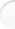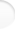Structure of Magic and Semi-Magic Squares, Methods and Tools for Enumeration by Francis GaspalouI give the listing of an example of enumeration program. This program is written in Basic for magic squares of order 3 (the algorithm is the same, whatever the order and the language). It is not optimized and has only a didactic interest.

The numbers 1 to 9 have to be put in a square. The magic constant is 15. It is well known that the number 5 is in the centre. Therefore, you need 2 parameters, for example A1=A and A2=B:

 A B 15-A-B 20-2*A-B 5 2*A+B-10 A+B-5 10-B 10-A

The principle of the algorithm is explained in the page Enumeration Programs, § 1.1.

 1. Program in Basic

10 REM MAGIC SQUARES OF ORDER 3
15 REM GENERAL ENUMERATION PROGRAM
20 REM 2 PARAMETERS A, B
25 CLS: DIM T(9): B2=5: T(5)=1
30:
35 REM FIRST PARAMETER A
40 FOR A=1 TO 9
45 A1=A: C3=10-A
50 IF T(A)=1 OR T(C3)=1 THEN 200
55 T(A)=1: T(C3)=1
60:
65 REM SECOND PARAMETER B
70 FOR B=1 TO 9
75 A2=B: C2=10-B: A3=15-A-B: C1=10-A3: B1=15-A-C1: B3=10-B1
80 IF A3< 1 OR A3> 9 OR B1< 1 OR B1> 9 THEN 190
85 IF T(B)=1 OR T(C2)=1 OR T(A3)=1 OR T(C1)=1 OR T(B1)=1 OR T(B3)=1 THEN 190
90 IF B=A3 OR B=C1 OR B=B1 OR B=B3 OR A3=B1 OR A3=B3 THEN 190
95:
100 REM RESULT
105 N=N+1
110 PRINT: PRINT N
115 PRINT A1,A2,A3
120 PRINT B1,B2,B3
125 PRINT C1,C2,C3
130:
190 NEXT B
195 T(A)=0: T(C3)=0
200 NEXT A

Result: 8 solutions

 2. Improvements Top of the pageFor B, you can calculate a lower boundary-stone IB (I as Inferior) and an upper boundary-stone SB (S as Superior) by conditions:
1< = A3 < =9  which give     1< = 15-A-B < =9     or       6-A < = B < =14-A
If you compare to 1< = B < =9, you have in Basic language:
If 1< 6-A then IB=6-A else IB=1
If 9< 14-A then SB=9 else SB=14-A

These boundary-stones IB and SB can be more improved if you write conditions 1< = B1 < =9.
You find:
1< = 20-2*A-B < =9       or       11-2*A < = B < = 19-2*A
In Basic, you create two new boundary-stones JB and TB:
If IB< 11-2*A then JB=11-2*A else JB=IB
If SB< 19-2*A then TB=SB else TB=19-2*A

Here is the improved program:

10 REM MAGIC SQUARES OF ORDER 3
15 REM GENERAL ENUMERATION PROGRAM
20 REM 2 PARAMETERS A, B
25 CLS: DIM T(9): B2=5: T(5)=1
30:
35 REM FIRST PARAMETER A
40 FOR A=1 TO 9
45 A1=A: C3=10-A
50 IF T(A)=1 OR T(C3)=1 THEN 200
55 T(A)=1: T(C3)=1
60:
65 REM SECOND PARAMETER B
66 IF 1< 6-A THEN IB=6-A: SB=9 ELSE IB=1: SB=14-A
67 IF IB< 11-2*A THEN JB=11-2*A ELSE JB=IB
68 IF SB< 19-2*A THEN TB=SB ELSE TB=19-2*A
69 IF JB> TB THEN 195

70 FOR B=JB TO TB
75 A2=B: C2=10-B: A3=15-A-B: C1=10-A3: B1=15-A-C1: B3=10-B1
80
85 IF T(B)=1 OR T(C2)=1 OR T(A3)=1 OR T(C1)=1 OR T(B1)=1 OR T(B3)=1 THEN 190
90 IF B=A3 OR B=C1 OR B=B1 OR B=B3 OR A3=B1 OR A3=B3 THEN 190
95:
100 REM RESULT
105 N=N+1
110 PRINT: PRINT N
115 PRINT A1,A2,A3
120 PRINT B1,B2,B3
125 PRINT C1,C2,C3
130:
190 NEXT B
195 T(A)=0: T(C3)=0
200 NEXT A

 3. Reduced Program Top of the pageIt should be possible to make also a "reduced program" (I call such a program a "reduced program/8") by application of group G of order 8.

The conditions should be for example:
A1 = minimum of {A1, A3, C1, C3} by vertical symmetry, horizontal symmetry and